# Math Solutions

5 Items found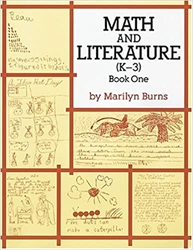Math and Literature (K-3) Book One by Marilyn Burns from Math Solutions for Adult in Math Reference & Teaching Aids (Location: MATR-TA) \$8.00 (1 in stock)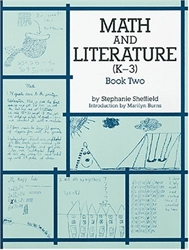Math and Literature (K-3) Book Two by Stephanie Sheffield, introduction by Marilyn Burns from Math Solutions for Adult in Math Reference & Teaching Aids (Location: MATR-TA) \$8.00 (1 in stock)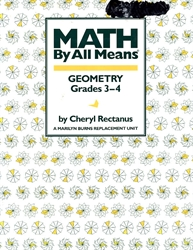Math By All Means: Geometry by Cheryl Rectanus from Math Solutions in Geometry & Shapes (Location: MATR-GEO)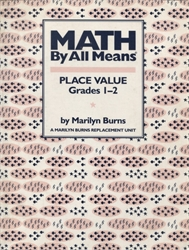Math By All Means: Place Value by Marilyn Burns from Math Solutions for 1st-2nd grade in Math Reference & Teaching Aids (Location: MATR-TA) \$6.00 (1 in stock)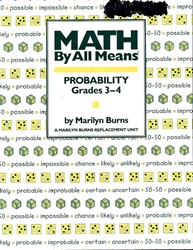Math By All Means: Probability by Marilyn Burns from Math Solutions in Math Games & Activities (Location: MATR-GAM)